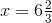# PSAT Math : How to find out a mixed fraction from an improper fraction

## Example Questions

### Example Question #11 : General Fractions

To make 48 cookies you need 1 cup white sugar, 1 cup packed brown sugar and 3 cups of flour.  You want to make 12 cookies, so you adjust the ingredients accordingly.  After the adjustment, how many total cups of dry ingredients do you have?

1 1/2 c

3/4 c

1 1/4 c

1 3/4 c

1/4 c

1 1/4 c

Explanation:

Going from 48 cookies to 12 cookies is a scaling factor of 1/4, so all the ingredients get multiplied by 1/4.  In order to make 12 cookies you will need 1/4 c white sugar, 1/4 c packed brown sugar, and 3/4 c flour.  Added all together you get 5/4 or 1 1/4 c.

### Example Question #2 : Mixed / Improper Fractions

What is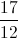written as a mixed number?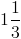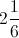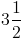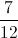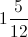Explanation:

How many times does 12 go into 17?  Once with a remainder of 5.

Sobecomes### Example Question #146 : Arithmetic

If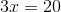, what doesequal?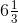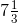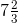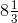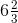Explanation:

First, you must solve the equation for. Then, you must convert the answer's improper fraction to a mixed fraction.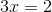Solve for.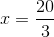Now, to convert an improper fraction, you must see how many times the denominator goes into the numerator, and then leave the remainder as a fraction. Example below:

Find out how many times the denominator goes into the numerator: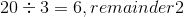Therefore,